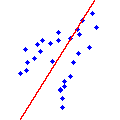CGAL 5.3 - Principal Component Analysis
Principal Component Analysis ReferencePierre Alliez, Sylvain Pion and Ankit Gupta
This package provides functions to compute global information about the shape of a set of 2D or 3D objects. It provides the computation of axis-aligned bounding boxes for point sets, and barycenters of weighted point sets. In addition, it provides computation of centroids (center of mass) and linear least squares fitting for point sets as well as for sets of other bounded objects. More specifically, it is possible to fit 2D lines to 2D segments, circles, disks, iso rectangles and triangles, as well as to fit 3D lines or 3D planes to 3D segments, triangles, iso cuboids, tetrahedra, spheres and balls. The common interface to these functions takes an iterator range of objects.
Introduced in: CGAL 3.2
Depends on: CGAL and Solvers
Windows Demos: Principal Component Analysis, Operations on Polygons, Polyhedron demo
Common Demo Dlls: dlls

## Functions

• CGAL::barycenter()
• CGAL::bounding_box()
• CGAL::centroid()
• CGAL::linear_least_squares_fitting_2()
• CGAL::linear_least_squares_fitting_3()

## Modules

CGAL::barycenter()
The function barycenter() computes the barycenter (weighted center of mass) of a set of 2D or 3D weighted points.

CGAL::bounding_box()

CGAL::centroid()
The function centroid() computes the (uniform) center of mass of a set of 2D or 3D bounded objects.

CGAL::linear_least_square_fitting_2()

CGAL::linear_least_square_fitting_3()